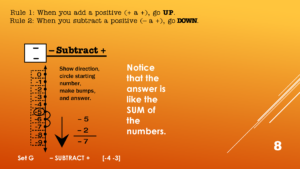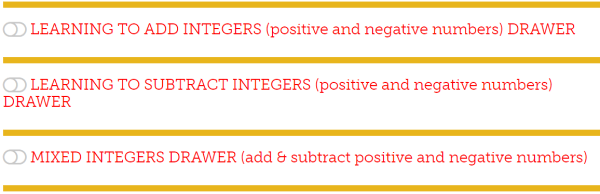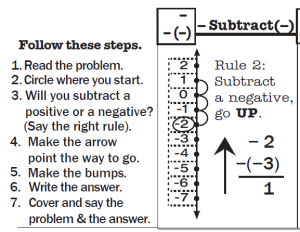## Are your students wary of working with integers?

Many students find integers confusing.  If you add a negative to a negative are you getting more or less??? Over the years different “rules” have been used to try to remember what should happen.  Rules such as “two negatives make a plus” or “opposite signs subtract.”  Whatever is used to try to remember, it interferes with a student’s ability to quickly and reliably get the answers without having to stop and puzzle it out.

##A Vertical Number line is more intuitively obvious for learning integers.

I have posted a series of free lessons online (links below) that use a vertical number line to take some of the confusion out of the process.  Turns out there are a total of eight types of problems but all of them can be solved with the same process on the vertical number line.  Intuitively on a vertical number line, up is more and down is less.

(1) Mixed Integers Set A1 Positive add a positive

(2) Mixed Integers Set A2 Positive subtract a positive

(3) Mixed Integers Set D Negative add a positive

(4) Mixed Integers Set G Negative subtract a positive

(5) Mixed Integers Set J Negative subtract a negative

(6) Mixed Integers Set M Positive subtract a negative

(7) Mixed Integers Set P Positive add a negative

(8) Mixed Integers Set S Negative add a negative

## Two rules to learn: when to go up and when to go down.

Using the vertical number line there are two rules to learn.  Rule 1: When you add a positive or subtract a negative you go up on the number line.  Rule 2: When you subtract a positive or add a negative you go down on the number line.

So the first thing to figure out is whether you’re going up or down.  Once you do that you simply make “bumps” going either up or down from where you start.  That gives you the answer without any uncertainty.  These lessons are quick (about 2 minutes) and identify a pattern of whether the answer is like the sum or the difference between the numbers.  Once students can recognize the pattern they can begin to answer fluently and without a struggle.

To help with the work of learning to quickly and easily recognize each pattern in Integers Rocket Math now includes three “Integers” Learning Tracks in our Worksheet Program Universal Level Subscription.    (Click here to get a 60-day initial trial subscription for less than the standard full-year subscription.)## Learn adding and subtracting separately or mixed.

The first integers learning track, Learning to Add Integers, is limited to adding integers.  In the above list of lessons, the adding lessons are numbers 1, 3, 7, and 8.  The second integers learning track, Learning to Subtract Integers, is limited to subtracting integers and teaches the processes shown in lessons 2, 4, 5, and 6.  The third learning track, Mixed Integers Drawer combines all 8 processes into one learning track.  If students are likely to have issues or begin to find the Mixed Integers lessons difficult, have them do the separate operation first.

In the Rocket Math Integers lessons, students use the vertical number line to work a problem.In this example: -2 minus (-3).  They use a rule and then apply it to the number line to find the answer. Then they have a set of problems with the same pattern they can orally answer without having to use the number line.

## Students practice with a peer partner

As with all Rocket Math programs, there is a 3-minute practice session, with a partner.  The partner who is checking has the answer key and corrects all errors immediately.  Then the two switch roles.  Then the practice is followed by a one-minute test.  If the student can answer the problems without hesitation the level is passed.  If it is still difficult the student stays at that level a bit longer.  When a new pattern is introduced the tests will have a whole row of problems that are the same pattern. When that level is passed the next test will have two types of problems in each row.  The next level has 3 types, then 4 types in each row.  Then the problem types are mixed.  This way the student develops fluency in recognizing the type of problem and how to derive the answer quickly.

Rocket Math has a money-back satisfaction guarantee.  If you try this and find it isn’t everything you hoped, for in terms of helping your students become fluent with integers, I’ll gladly refund your money.  I’m betting they’re going to love it.